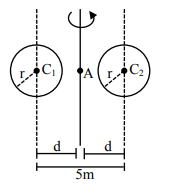# A system consists of two identical spheres each of mass`
Question:

A system consists of two identical spheres each of mass $1.5 \mathrm{~kg}$ and radius $50 \mathrm{~cm}$ at the end of light rod. The distance between the centres of the two spheres is $5 \mathrm{~m}$. What will be the moment of inertia of the system about an axis perpendicular to the rod passing through its midpoint?

1. $18.75 \mathrm{kgm}^{2}$

2. $1.905 \times 10^{5} \mathrm{kgm}^{2}$

3. $19.05 \mathrm{kgm}^{2}$

4. $1.875 \times 10^{5} \mathrm{kgm}^{2}$

Correct Option: , 3

Solution:$\mathrm{M}=1.5 \mathrm{~kg}, \mathrm{r}=0.5 \mathrm{~m}, \mathrm{~d}=\frac{5}{2} \mathrm{~m}$

$\mathrm{I}=2\left(\frac{2}{5} \mathrm{Mr}^{2}+\mathrm{Md}^{2}\right)$

$=19.05 \mathrm{kgm}^{2}$